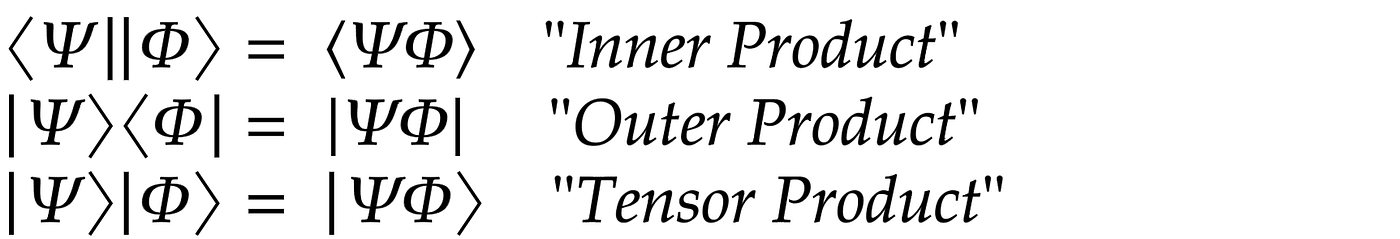# Qubit, An Intuition #3 — Quantum Measurement, Full and Partial Qubits

`TL;DR; Quantum measurement for full and partial qubits, with examples. Quantum state. Pure state.Please refer to the previous articles:“Qubit, An Intuition #1 — First Baby Steps in Exploring the Quantum World” for a discussion on a single qubit as a computing unit for quantum computation.- "Qubit, An Intuition #2 - Inner Product, Outer Product, and Tensor Product" for a discussion on two-qubits operations.`

# Qubit and Two-Qubits operations

A single quantum bit (qubit) is exciting with its nature of being in superposition. Hence |Ψ> = α|0> + β|1>. The quantum state Psi is in the linear combination of |0> and |1> with the probability of being in state |0> is |α|², and the probability of being in state|1> is |β|².Bra-ket notation for two-qubit operations: inner product, outer product, and tensor product.

# The Quantum States & Pure States

A few rules in quantum measurements:

• First, following a measurement, a qubit (or qubits) in a superposition state switches to a pure state. It means the qubits lost their quantumness.
• Second, the new resultant state must always preserve the normalization constraint.

# Full Qubits Quantum Measurement

Let’s take an example of a two-qubits state |Ψ>. Note that there are only three linear combinations of states |00>, |10>, and |11>.

# Partial Qubits Quantum Measurement

Let’s use the same two-qubits state |Ψ> to illustrate partial qubits measurement.

## Example 1 — Partial measurement of 2 Qubits State

The following is the measurement of the first qubit to get the first qubit = 1. To do this, we only need to pay attention to the |10> and |11> states, which have the first qubit = 1 (highlighted below).

• After measurement, the measured qubits changed from superposition state to pure state.
• The new resultant state must always preserve the normalization constraint. Thus, it must be renormalized.

## Example 2 —Partial measurement of 2 Qubits State

Let’s use another example for the same two-qubits state |Ψ> to illustrate a different partial qubits measurement.

## Example 3 — Partial measurement of 4 Qubits State

Let’s use one more example for the four-qubits state |Ψ> to illustrate a different partial qubits measurement.

# Moving Forward

Next in the “Qubit, An Intuition #4” article, we will discuss unitary matrices, with examples and their implementation in IBM quantum.

--

--